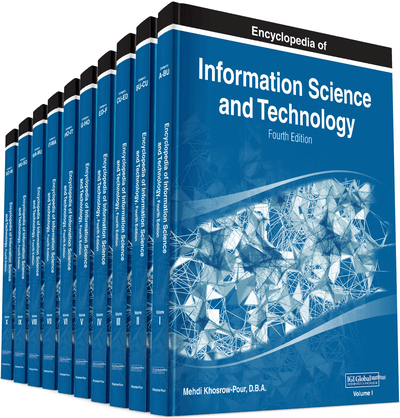# Uniform Random Number Generation With Jumping Facilities

E. Jack Chen (BASF Corporation, USA)
DOI: 10.4018/978-1-5225-2255-3.ch111

## Abstract

A facility for generating sequences of pseudorandom numbers is a fundamental part of computer simulation systems. Furthermore, multiple independent streams of random numbers are often required in simulation studies, for instance, to facilitate synchronization for variance-reduction purposes, and for making independent replications. A portable set of software utilities is described for uniform random-number generation. It provides for multiple generators (streams) running simultaneously, and each generator (stream) has its sequence of numbers partitioned into many long disjoint contiguous substreams. Simple procedure calls allow the user to make any generator “jump” ahead/back v steps (random numbers). Implementation issues are discussed. An efficient and portable code is also provided to implement the package. The basic underlying generator CMRG (combined Multiple Recursive Generator) combines two multiple recursive random number generators with a period length of approximately 2191 (˜ 3.1× 1057), good speed, and excellent theoretical properties.
Chapter Preview
Top

## Background

There are a number of methods for generating the random numbers, of which the most popular are the congruential methods (mixed, multiplicative, and additive). The (mixed) linear congruential generators (LCGs) are defined by­x0∈{1,…,m–1} i > 0.where m (the modulus) is a positive integer (usually a very large primary number), a (the multiplier) ∈{1,…,m–1} and c (the increment) is a nonnegative integer. This mathematical notation signifies that xi is the remainder of (axi-1+c) divided by m. Hence, xi∈{1,…,m–1}. Thus, random variable ui is a uniform 0, 1 variable. Note that

.

## Key Terms in this Chapter

Quantile Function (Inverse Distribution Function): The quantile function (given a probability p ) returns the value at or below which 100 p percent of the population lies.

Common Random Numbers: A variance reduction technique that uses the same random number streams to simulation different systems.

Deterministic Algorithm: An algorithm that given the same input always products the same outputs.

Random Number Generators (RNGs): Computational or physical devices designed to generate a sequence of numbers that appear to be random, as determined by statistical tests.

Deterministic Sequence: A sequence that the value of any future elements can be determined when the value of the current element is known

Jump Ahead (Back) v Steps: A utility to advance (go back) v steps in the random number stream without going through the stream sequentially.

Period Length (of RNGs): The length of the random number sequence before it recycles itself.

Variance Reduction: A technique to reduce the variance of the estimate, hence, increase the precision of the estimate.

## Complete Chapter List

Search this Book:
Reset